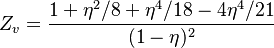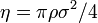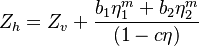2022: SklogWiki celebrates 15 years on-line

Difference between revisions of "Liu hard disk equation of state"$Z_v = \frac{1 + \eta^2/8 + \eta^4/18 - 4 \eta^4/21}{(1-\eta)^2}$
where the packing fraction is given by$\eta = \pi \rho \sigma^2 /4$ where$\sigma$ is the diameter of the disks.$Z_h = Z_v + \frac{b_1 \eta^m_1 + b_2 \eta^m_2}{(1-c \eta)}$